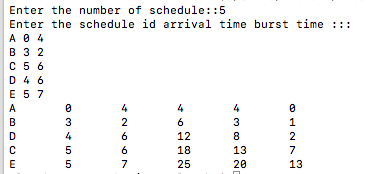# Implementation of Shortest Job First (SJF) Non-Preemptive CPU scheduling algorithm using C++

In this article, we are going to learn about implementation of shortest job first (SJF) Non-preemptive scheduling algorithm using C++ program.
Submitted by Aleesha Ali, on January 26, 2018

Non-preemptive: We cannot remove a process until it completes it execution.

Scheduling criteria tells us that any algorithm is how much efficient, the main criteria of scheduling are given below:

• CPU Utilization
• Throughput
• Arrival time
• Turn around time
• Waiting time
• Completion time
• Burst time

*Ready Queue is a queue where all the processes wait to get CPU for its execution.

CPU Utilization: The amount of time CPU is busy.

Throughput: The number of process computed per unit time.

Arrival time: The time at which the process enters into ready queue.

Turn around time: The interval between the time of submission of a process to the time of completion.

Waiting time: The total amount of the time a process spends in ready queue.

Completion time: The time at which process completes its execution.

Burst time: The time needed by CPU to completes its execution.

## Shortest Job First Algorithm

In this scheduling algorithm the process having minimum burst time will execute first.

### C++ Program for SJF scheduling

```//Implementation fo SHORTEST JOB FIRST Using C++

#include <iostream>
#include <algorithm>
using namespace std;

int ab;

typedef struct schedule
{
string pro_id;
int at,bt,ct,ta,wt;

/*
artime = Arrival time,
bt = Burst time,
ct = Completion time,
ta = Turn around time,
wt = Waiting time
*/

}schedule;

bool compare(schedule a,schedule b)
{
return a.at < b.at;
/* This process will always return TRUE
if above condition comes*/
}

bool compare2(schedule a,schedule b)
{
return a.bt < b.bt && a.at <= ab;
/* This process will always return TRUE
if above condition comes*/
}

int main()
{
schedule pro;
//An array of Processes
int n,i,j;
//n = number of processes, i= iteration variable

cout<<"Enter the number of schedule::";
cin>>n;
cout<<"Enter the schedule id arrival time burst time :::";

for(i=0;i<n;i++)
{
cin>>pro[i].pro_id;
cin>>pro[i].at;
cin>>pro[i].bt;
}

/*sort is a predefined funcion  defined in algorithm.h header file,
it will sort the processes according to their arrival time*/

sort(pro,pro+n,compare);

// initial values

pro.ct=pro.bt+pro.at;
pro.ta=pro.ct-pro.at;
pro.wt=pro.ta-pro.bt;

for(i=1;i<n;i++)
{
ab=pro[i-1].ct;
sort(pro+i,pro+n,compare2);
if(pro[i-1].ct<pro[i].at)
{
pro[i].ct=pro[i-1].ct+pro[i].bt+(pro[i].at-pro[i-1].ct);
}
else
{

pro[i].ct=pro[i-1].ct+pro[i].bt;

}
pro[i].ta=pro[i].ct-pro[i].at;
pro[i].wt=pro[i].ta-pro[i].bt;
}

for(i=0;i<n;i++)
{
//before executing make it in one statement
cout<<pro[i].pro_id<<"\t"<<pro[i].at<<"\t"<<pro[i].bt
<<"\t"<<pro[i].ct<<"\t"<<pro[i].ta<<"\t"<<pro[i].wt;

cout<<endl;
}

return 0;
}
```

OutputWhat's New (MCQs)

Top Interview Coding Problems/Challenges!

Languages: » C » C++ » C++ STL » Java » Data Structure » C#.Net » Android » Kotlin » SQL
Web Technologies: » PHP » Python » JavaScript » CSS » Ajax » Node.js » Web programming/HTML
Solved programs: » C » C++ » DS » Java » C#
Aptitude que. & ans.: » C » C++ » Java » DBMS
Interview que. & ans.: » C » Embedded C » Java » SEO » HR
CS Subjects: » CS Basics » O.S. » Networks » DBMS » Embedded Systems » Cloud Computing
» Machine learning » CS Organizations » Linux » DOS
More: » Articles » Puzzles » News/Updates

© https://www.includehelp.com some rights reserved.